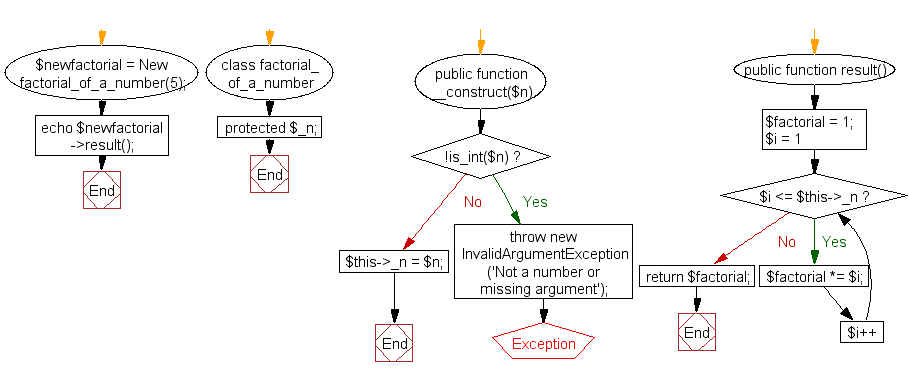﻿ PHP Class Exercise: Calculate the factorial of an integer - w3resource# PHP Class Exercises : Calculate the factorial of an integer

## PHP class: Exercise-3 with Solution

Write a PHP class that calculates the factorial of an integer.

Sample Solution:

PHP Code:

``````<?php
class factorial_of_a_number
{
protected \$_n;
public function __construct(\$n)
{
if (!is_int(\$n))
{
throw new InvalidArgumentException('Not a number or missing argument');
}
\$this->_n = \$n;
}
public function result()
{
\$factorial = 1;
for (\$i = 1; \$i <= \$this->_n; \$i++)
{
\$factorial *= \$i;
}
return \$factorial;
}
}

\$newfactorial = New factorial_of_a_number(5);
echo \$newfactorial->result();
?>
```
```

Sample Output:

```120
```

Flowchart :PHP Code Editor:

Have another way to solve this solution? Contribute your code (and comments) through Disqus.

What is the difficulty level of this exercise?

﻿

## PHP: Tips of the Day

Get first key in a (possibly) associative array?

Starting from PHP 7.3, there is a new built in function called array_key_first() which will retrieve the first key from the given array without resetting the internal pointer. Check out the documentation for more info.

You can use reset and key:

```reset(\$array);
\$first_key = key(\$array);
```

It's essentially the same as your initial code, but with a little less overhead, and it's more obvious what is happening.

Just remember to call reset, or you may get any of the keys in the array. You can also use end instead of reset to get the last key.

If you wanted the key to get the first value, reset actually returns it:

`\$first_value = reset(\$array);`

There is one special case to watch out for though (so check the length of the array first):

```\$arr1 = array(false);
\$arr2 = array();
var_dump(reset(\$arr1) === reset(\$arr2)); // bool(true)
```

Ref : https://bit.ly/32ecxHN